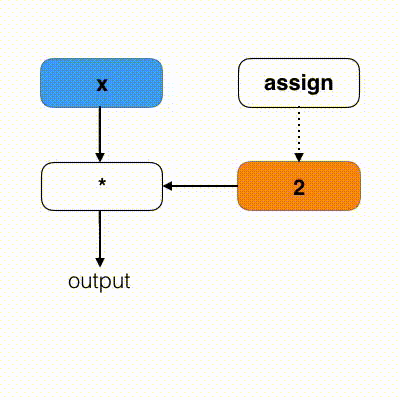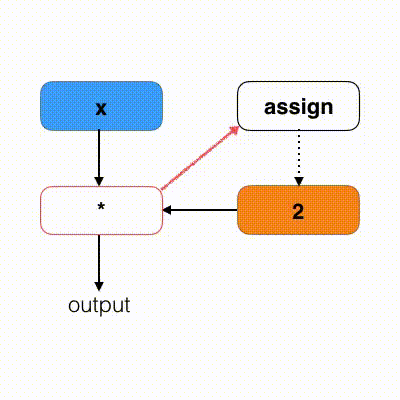# 目录

TensorFlow学习系列（一）：初识TensorFlow

TensorFlow学习系列（二）：形状和动态维度

TensorFlow学习系列（三）：保存/恢复和混合多个模型

TensorFlow学习系列（四）：利用神经网络实现泛逼近器（universal approximator）

TensorFlow学习系列（五）：如何使用队列和多线程优化输入管道

TensorFlow学习系列（六）：变量更新和控制依赖

# 变量更新

``````import tensorflow as tf

# We define a Variable
x = tf.Variable(0, dtype=tf.int32)

# We use a simple assign operation
assign_op = tf.assign(x, x + 1)

with tf.Session() as sess:
sess.run(tf.global_variables_initializer())

for i in range(5):
print('x:', sess.run(x))
sess.run(assign_op)

# outputs:
# x: 0
# x: 1
# x: 2
# x: 3
# x: 4``````

TF 有许多的函数来支持手动更新变量，你可以在 TensorFlow 的函数帮助页面进行查看，很多的操作都可以被一些张量操作来取代，然后调用 `tf.assign` 函数来实现更新操作，但在一些情况下，这将会是非常麻烦的一件事。所以，TensorFlow 为我们提供了两种更新操作：

• `tf.Variable` 函数中有一个参数 `validate_shape` 默认是设置为 `True` 。它阻止你对参数进行维度更新，所以我们必须将这个参数设置为 `False`

• 这个参数也存在于 `tf.assign` 函数中，所以我们也必须将这个参数进行关闭。

``````import tensorflow as tf

# We define a "shape-able" Variable
x = tf.Variable(
[], # A list of scalar
dtype=tf.int32,
validate_shape=False, # By "shape-able", i mean we don't validate the shape so we can change it
trainable=False
)
# I build a new shape and assign it to x
concat = tf.concat([x, ], 0)
assign_op = tf.assign(x, concat, validate_shape=False) # We force TF, to skip the shape validation step

with tf.Session() as sess:
sess.run(tf.global_variables_initializer())

for i in range(5):
print('x:', sess.run(x), 'shape:', sess.run(tf.shape(x)))
sess.run(assign_op)

# outputs:
# x: [] shape: 
# x:  shape: 
# x: [0 0] shape: 
# x: [0 0 0] shape: 
# x: [0 0 0 0] shape: ``````

# 控制依赖

``````import tensorflow as tf

# We define our Variables and placeholders
x = tf.placeholder(tf.int32, shape=[], name='x')
y = tf.Variable(2, dtype=tf.int32)

# We set our assign op
assign_op = tf.assign(y, y + 1)

# We build our multiplication (this could be a more complicated graph)
out = x * y

with tf.Session() as sess:
sess.run(tf.global_variables_initializer())

for i in range(3):
print('output:', sess.run(out, feed_dict={x: 1}))

# outputs:
# output: 2
# output: 2
# output: 2``````

``````import tensorflow as tf

# We define our Variables and placeholders
x = tf.placeholder(tf.int32, shape=[], name='x')
y = tf.Variable(2, dtype=tf.int32)

# We set our assign op
assign_op = tf.assign(y, y + 1)

# We build our multiplication, but this time, inside a control depedency scheme!
with tf.control_dependencies([assign_op]):
# Now, we are under the dependency scope:
# All the operations happening here will only happens after
# the "assign_op" has been computed first
out = x * y

with tf.Session() as sess:
sess.run(tf.global_variables_initializer())

for i in range(3):
print('output:', sess.run(out, feed_dict={x: 1}))

# outputs:
# output: 3
# output: 4
# output: 5``````

• 在上图，图并不会去计算 `assign_op`

• 在下图，控制依赖在计算乘法之前会强制图去计算 `assign_op`# 一个陷阱

``````import tensorflow as tf

# I define a "shape-able" Variable
x = tf.Variable(
[],
dtype=tf.int32,
validate_shape=False, # By "shape-able", i mean we don't validate the shape
trainable=False
)
# I build a new shape and assign it to x
concat = tf.concat([x, ], 0)
assign_op = tf.assign(x, concat, validate_shape=False)

with tf.control_dependencies([assign_op]):
# I print x after the assignment
x = tf.Print(x, data=[x, x.read_value()], message="x, x_read:")
# The assign_op is called, but it seems that print statement happens
# before the assignment, that is wrong.

with tf.Session() as sess:
sess.run(tf.global_variables_initializer())
for i in range(3):
sess.run(x)

# outputs:
# x: []   , x_read:  
# x:   , x_read:  [0 0]
# x: [0 0], x_read:  [0 0 0]``````

• 打印操作依赖于 `assign_op` ，它只能在 `x` 被更新之后计算。

• 然而，当我们打印 `x` 的时候，它看起来好像没有更新。

• 但实际上，由于我们可以使用特殊的 `read_value` 函数来获取 `x` 的真正值。

# Reference：

http://stackoverflow.com/questions/38994037/tensorflow-while-loop-for-training

https://github.com/tensorflow/tensorflow/issues/7782### AllenOR灵感

ApacheCN 人工智能知识树 v1.0

Special Sponsors 贡献者：飞龙 版本：v1.0 最近总是有人问我，把 ApacheCN 这些资料看完一遍要用多长时间，如果你一本书一本书看的话，的确要用很长时间。但我觉得这是非常麻烦的，因为每本...

ApacheCN_飞龙
05/18
0
0
TensorFlow 高效编程

TensorFlow 高效编程 原文：vahidk/EffectiveTensorflow 译者：FesianXu、飞龙 协议：CC BY-NC-SA 4.0 一、TensorFlow 基础 TensorFlow 和其他数字计算库（如 numpy）之间最明显的区别在于 ...

ApacheCN_飞龙
2018/07/11
0
0

2018/09/26
0
0
TensorFlow 2.0 的核心功能将是“Eager Execution”

TensorFlow 2.0 是谷歌开源机器学习框架的下一个主要版本，将于 2018 年末推出其首个测试版。TensorFlow 是 Google 对机器学习和数据科学领域的贡献，是快速开发神经网络的一般框架。 尽管 ...

2018/08/22
4K
5

【方向】
2018/08/25
0
0

Spring Boot + Mybatis-Plus 集成与使用（二）

7
0

@[TOC](用最通俗的方法讲spring [一] ──── AOP) 写这个系列的目的(可以跳过不看) 自己写这个系列的目的,是因为自己是个比较笨的人,我曾一度怀疑自己的智商不适合干编程这个行业.因为在我...

7
0
Flutter系列之在 macOS 上安装和配置 Flutter 开发环境

6
0
OSChina 周六乱弹 —— 早上儿子问我他是怎么来的

Osc乱弹歌单（2019）请戳（这里） 【今日歌曲】 @凉小生 ：#今日歌曲推荐# 少点戾气，愿你和这个世界温柔以待。中岛美嘉的单曲《僕が死のうと思ったのは (曾经我也想过一了百了)》 《僕が死の...

2.5K
16
Excption与Error包结构，OOM 你遇到过哪些情况，SOF 你遇到过哪些情况

Throwable 是 Java 中所有错误与异常的超类，Throwable 包含两个子类，Error 与 Exception 。用于指示发生了异常情况。 Java 抛出的 Throwable 可以分成三种类型。 被检查异常（checked Exc...

Garphy

42
0